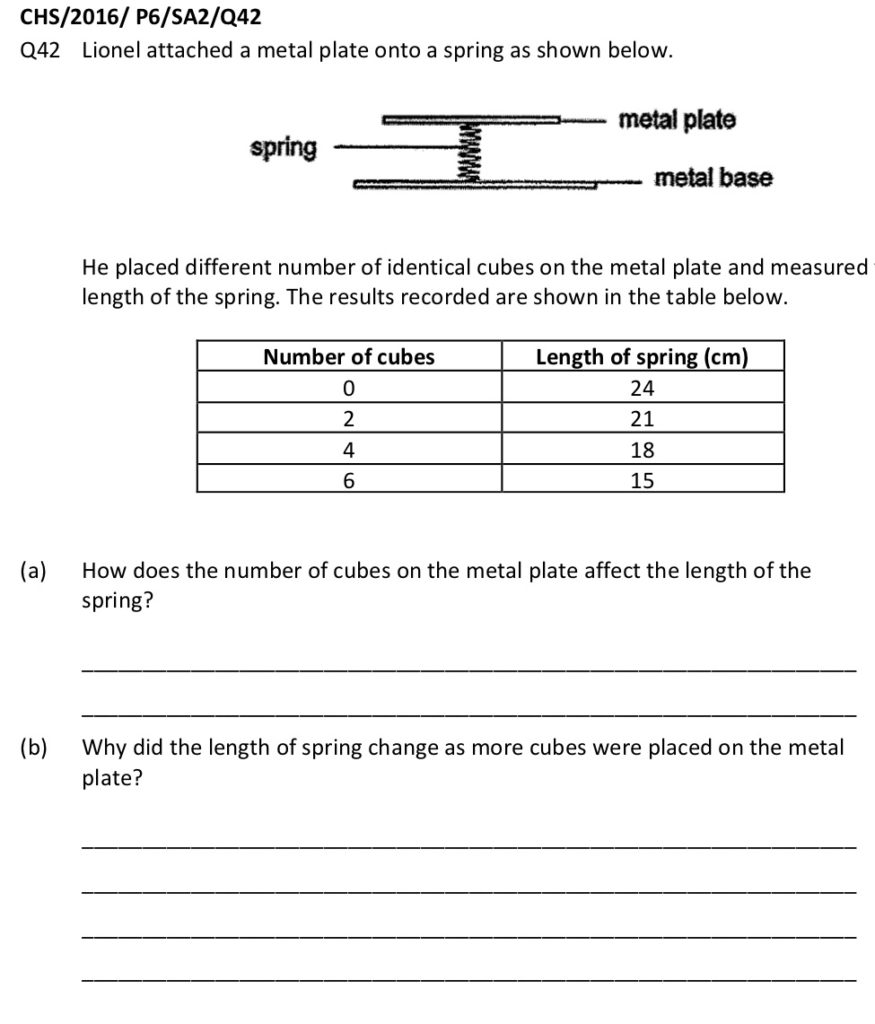Last Updated On

Springs can be found in many objects that we use in our daily lives. Are you able to name me a few objects that require a spring to function?

Were you able to mention any of the following objects?

1. Pens
2. Weighing scale/Spring balance
3. Vehicles (suspension)
4. Watches
5. Switches/Buttons

You may be wondering how these objects are related to what we learn in the primary school Science syllabus.

Springs are typically introduced when the Primary 6 topics of Energy and Forces are being taught. Some schools teach the aforementioned topics in Primary 5 instead.

From my observation, students usually do not have issues understanding the key concepts of Forces. However, it is the application of these concepts that cause students to encounter difficulties.

## What You Need To Know About Elastic Spring Force

To keep things simple in this article, I will be focusing on elastic spring force instead of elastic potential energy. Elastic spring force is the force acting on a stretched/compressed elastic object to return it to its original length.

Firstly, let us recall the 2 factors that affect elastic spring force:

Factor 1: The extent to which (how much) the elastic object is stretched or compressed.

• An elastic object that is more stretched or compressed would have more elastic spring force acting on it.
• An elastic object that is neither stretched or compressed would NOT have elastic spring force acting on it.

Factor 2: The stiffness of an object.

• A less stiff object can be stretched or compressed more easily.
• Comparing two elastic objects, more elastic spring force would act on the stiffer elastic object when they are stretched or compressed by the same length.

## Let Us Try This Experiment!

Now, I would like you to take the spring out from your ball point pen. Hold it using your index finger and your thumb as shown in the diagram below.What did you observe? Did the spring extend on its own?

The answer is no! The spring does not stretch on its own.

However, if you release the spring from your fingers, what will happen to the spring? The spring will fall to the ground.

How can we explain the above observation?

The gravitational force acting on the spring does not cause the spring to stretch.

However, the gravitational force acting on the spring would cause the spring to move downwards and fall to the ground.

## How Does The Spring Change When There Is A Load?A 1kg load is hung on the spring, causing the spring to stretch.

How do we then explain this observation?

There are 3 steps that are required in the answer.

1. The gravitational force acting on the load (and not the spring)
2. exerts a pull force on the spring,
3. causing the spring to stretch.

## What If The Spring Does Not Stretch With A Load?

Given another scenario, what happens when we attach a 1kg load to a spring and the spring does not stretch? Are you able to explain this concept in a scientific manner?Here is an answer suggested by one of my students:

The gravitational force acting on the load (downward force) was not able to overcome elastic spring force acting on the spring (upward force).

What do you think of my student’s answer above?

Let us recall that there will only be an elastic spring force acting on a spring when it is stretched or compressed, as mentioned earlier in this article. Since the gravitational force acting on the load was unable to cause the spring to stretch, the spring would not have any elastic spring force acting on it.

The gravitational force acting on the load was insufficient/not strong enough to cause the spring to stretch.

With this understanding of elastic spring force, we will now attempt an examination question using the concepts discussed.

## Let’s Take A Look At This Question HereSource: Catholic High School – 2016 P6 Preliminary Science Examination Paper [Q42]

## Tackling Part (A) Of The Above Question

The 2 variables in this question are “the number of cubes on the metal plate” and “the length of the spring”.

Hence, we need to ask ourselves:

“Which is the changed variable?” and “Which is the measured variable?”

Since the “number of cubes on the metal plate” is the changed variable, the changed variable should come first.

As the number of cubes on the metal plate increases, the length of the spring decreases.

## Tackling Part (B) Of The Above Question

As more cubes are placed on the metal plate, the mass of the cubes on the metal plate increases. Thus, the gravitational force acting on the cubes increases, which increases the push force exerted downwards on the spring balance. This causes the spring to be compressed more.

Since the spring is now compressed, there will be elastic spring force acting on the spring (upwards) to return the spring to its original length. As such, we will now be able to mention elastic spring force in our answers.